# To calculate:The ratio of line AB and BC. Given information: The following diagram is given 12210203181.jpgQuestion
SimilarityTo calculate:The ratio of line AB and BC.
Given information:
The following diagram is given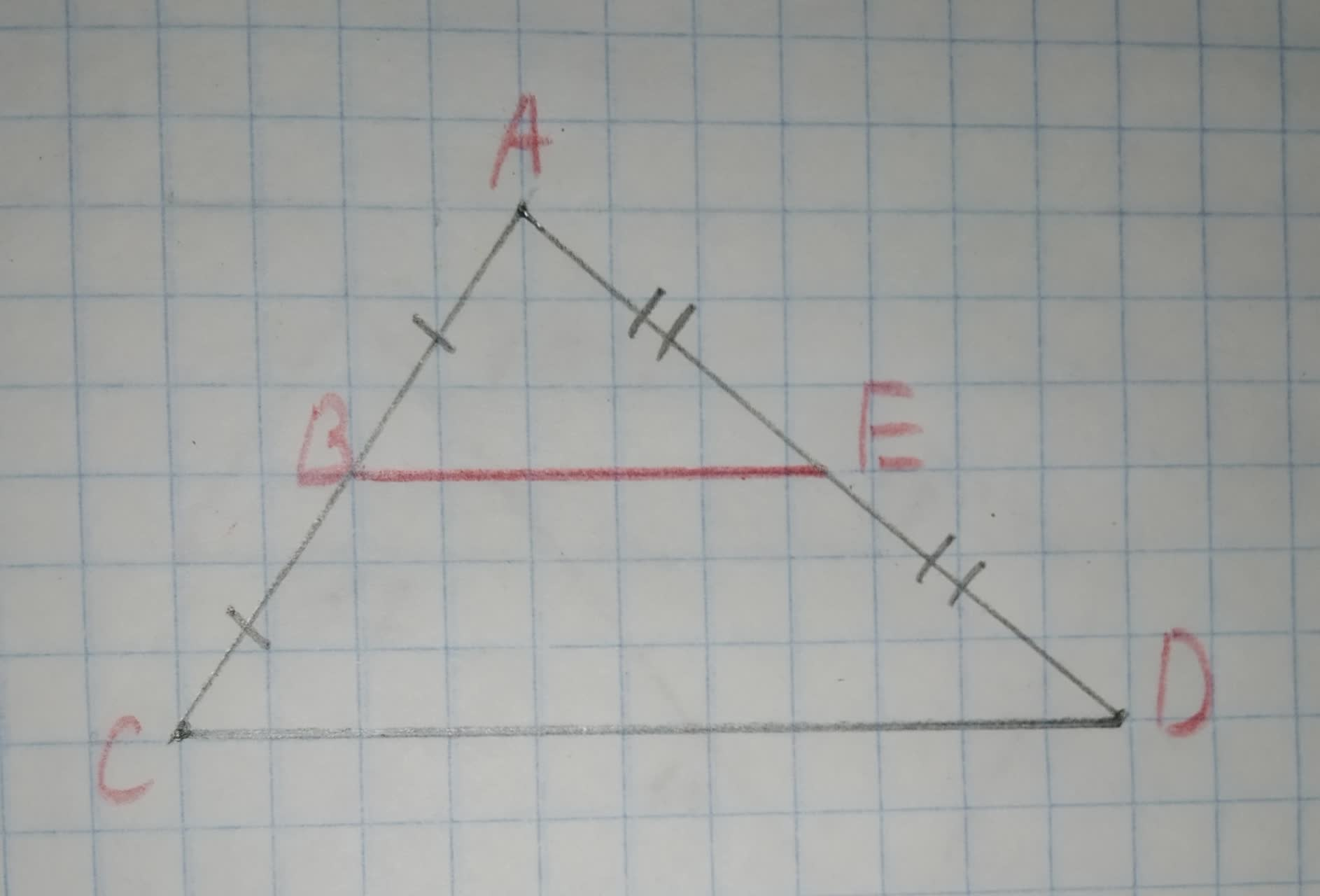2020-11-02
Formula used AAA similarity criterion
Calculation: $$\displaystyle\triangle{A}{B}{E}&\triangle{A}{C}{D}$$ are similar through AAA similarity.
Therefore,
AB=BC
Therefore, the ratio of AB and BC will be
$$\displaystyle\therefore$$Ratio of AB and AC is 1:1

### Relevant QuestionsTo calculate:The ratio of line AB and AC.
Given information:To check: whether the additional information in the given option would be enough to prove the given similarity.
Given:
The given similarity is $$\displaystyle\triangle{A}{D}{C}\sim\triangle{B}{E}{C}$$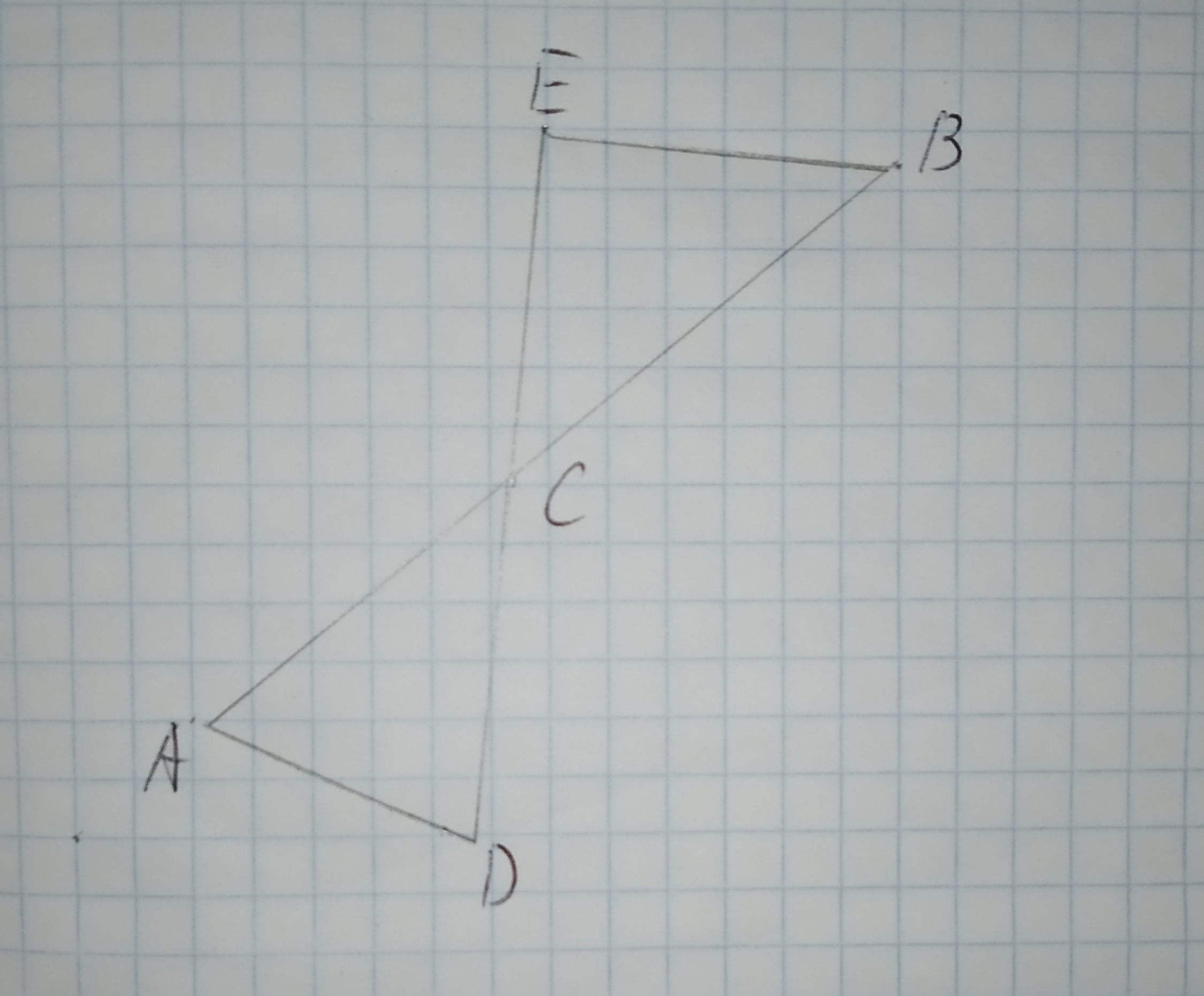The given options are:
A.$$\displaystyle\angle{D}{A}{C}{\quad\text{and}\quad}\angle{E}{C}{B}$$ are congruent.
B.$$\displaystyle\overline{{{A}{C}}}{\quad\text{and}\quad}\overline{{{B}{C}}}$$ are congruent.
C.$$\displaystyle\overline{{{A}{D}}}{\quad\text{and}\quad}\overline{{{E}{B}}}$$ are parallel.
D.$$\displaystyle\angle{C}{E}{B}$$ is a right triangle.To determine:To Find:the measure of side BB' in the figure shown such that VA' = 15, A'A=20 and A'B'=24
Given:
Figure is shown below.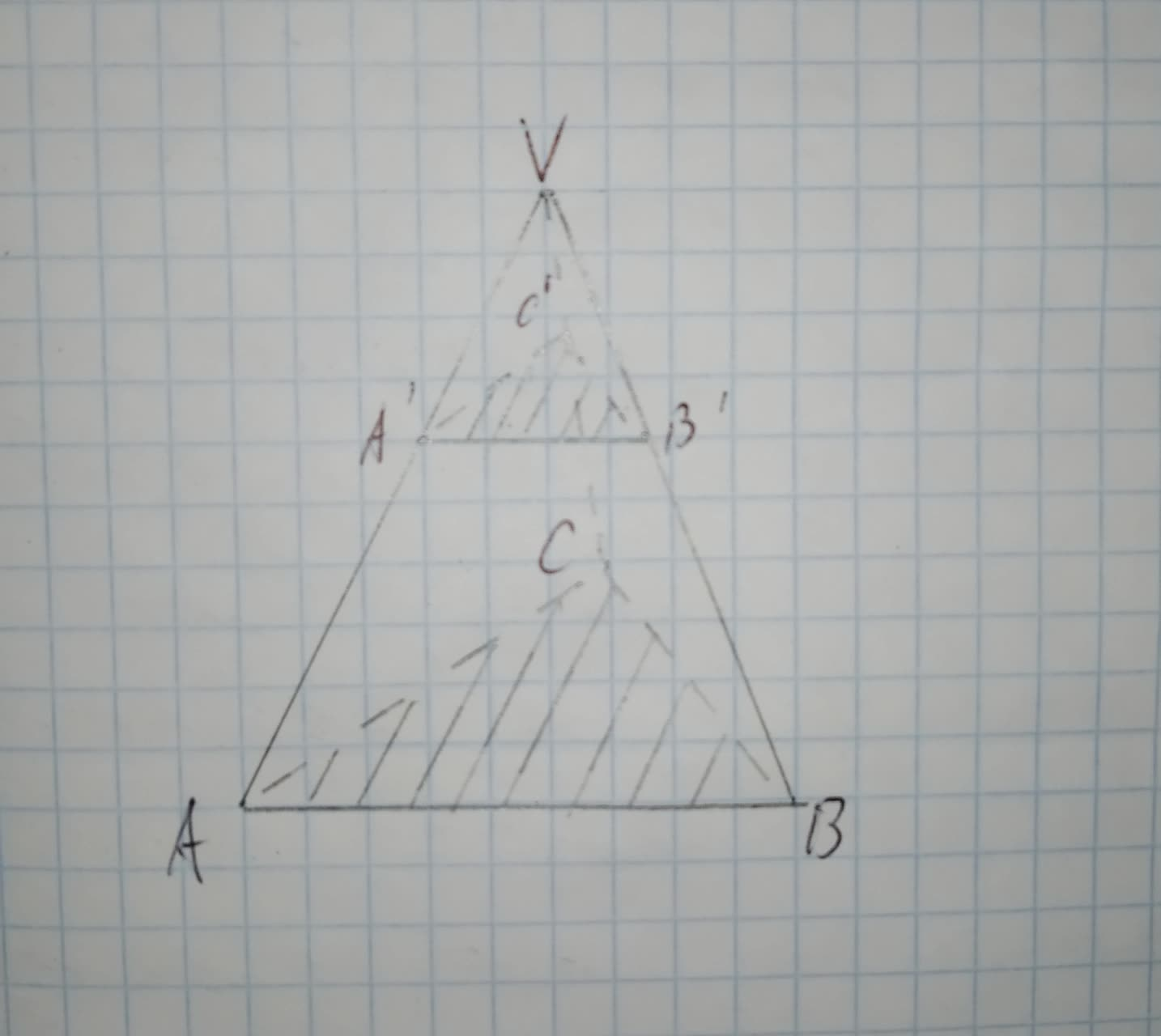To write: the name of the postulate, that justifies the given statement.
Given information:In $$\displaystyle\triangle{A}{V}{B}{\quad\text{and}\quad}\triangle{N}{V}{K},\angle{A}=\angle{N}={60}^{{\circ}}$$.
The given figure is as follows: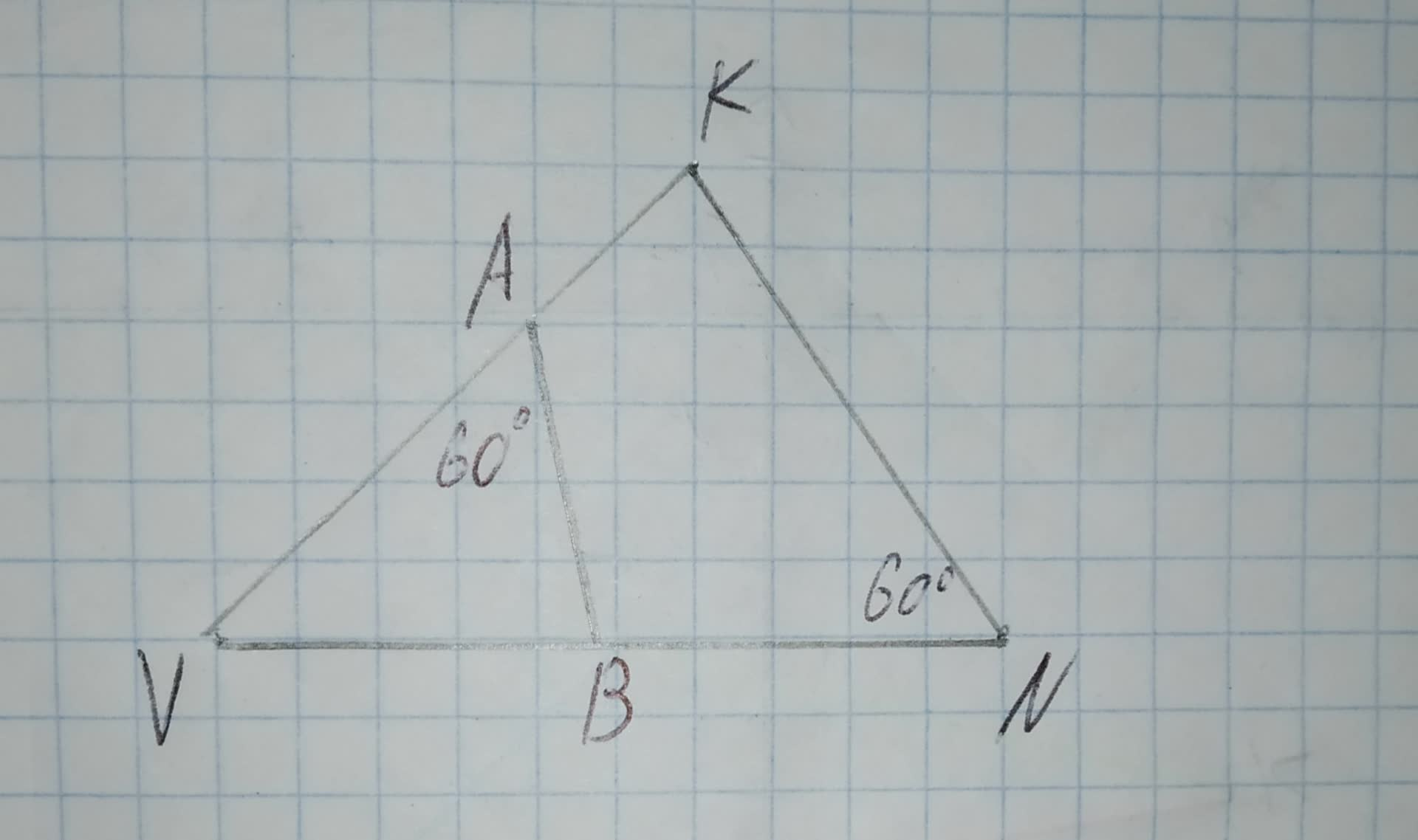To determine:Whether each pair of figures is similar and also write similarity statement and scale factor.
Given information:
Given the figure.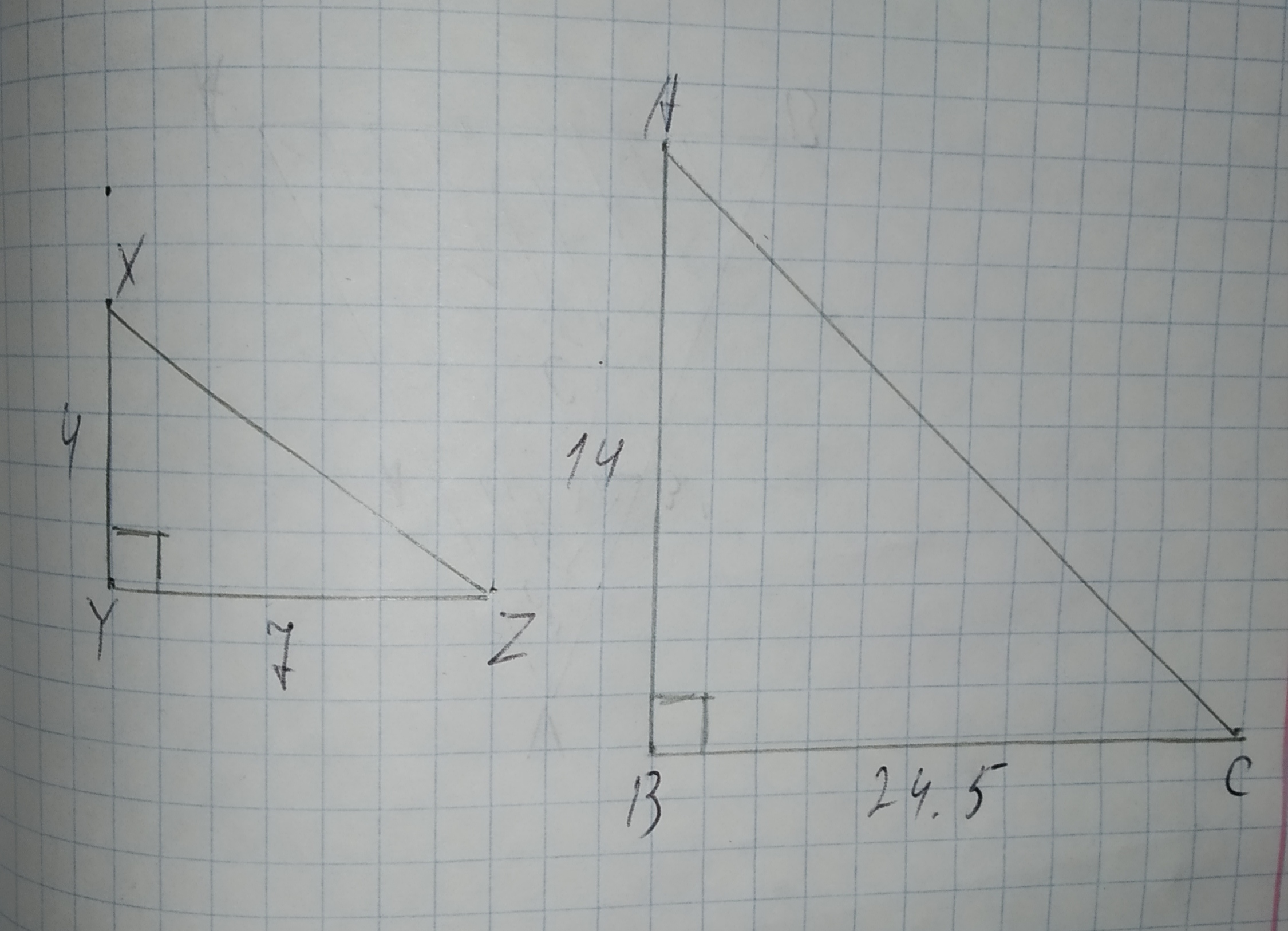Given $$\displaystyle\triangle{D}{E}{F}{\quad\text{and}\quad}\triangle{E}{G}{F}$$ in the diagram below, determine if the triangles are similar. If so, write a similarity statement, and state the criterion used to support your claim.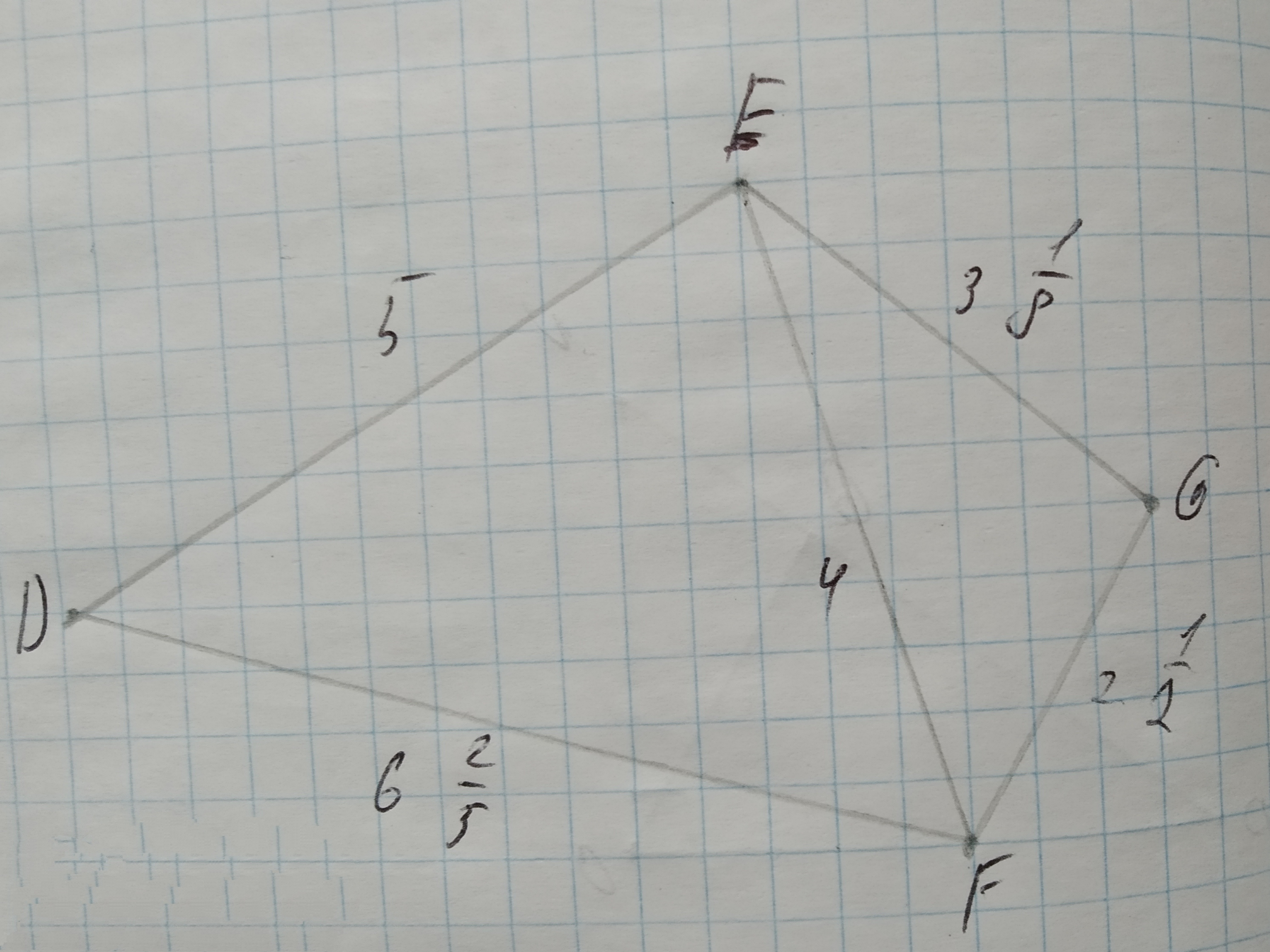Determine whether $$\displaystyle\triangle{A}{B}{C}{\quad\text{and}\quad}\triangle{D}{E}{F}$$ are similar for each set of measures. If so, identify the similarity criterion.
1.$$\displaystyle{m}\angle{A}={20},{m}\angle{C}={20},{m}\angle{D}={40},{m}\angle{F}={40}$$
2.$$\displaystyle{m}\angle{A}={20},{m}\angle{C}={4}{u},{m}\angle{D}={20},{m}\angle{F}={40}$$
3.$$\displaystyle{A}{B}={20},{B}{C}={40},{m}\angle{B}={53}$$,
$$\displaystyle{D}{E}={10},{E}{F}={20},{m}\angle{E}={53}$$To prove:The congruency of $$\displaystyle\vec{{{M}{H}}}\stackrel{\sim}{=}\vec{{{J}{O}}}$$.
Given information:
The following information has been given GJKM is a rhombus
$$\displaystyle\angle{J}{O}{G}=\angle{M}{H}{G}={90}^{{\circ}}$$To prove:The congruency of $$\displaystyle\vec{{{S}{O}}}\stackrel{\sim}{=}\vec{{{T}{O}}}$$.
$$\displaystyle\angle{S}{O}{V}=\angle{T}{O}{W}$$
$$\displaystyle\angle{W}{S}{O}=\angle{V}{T}{O}$$To prove: The congruency of $$\displaystyle\triangle{R}{K}{M}\stackrel{\sim}{=}\triangle{O}{M}{K}$$.
$$\displaystyle\angle{M}{R}{K}=\angle{K}{O}{M}={90}^{{\circ}}$$
$$\displaystyle\angle{R}{K}{M}=\angle{O}{M}{K}$$